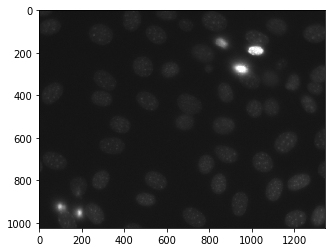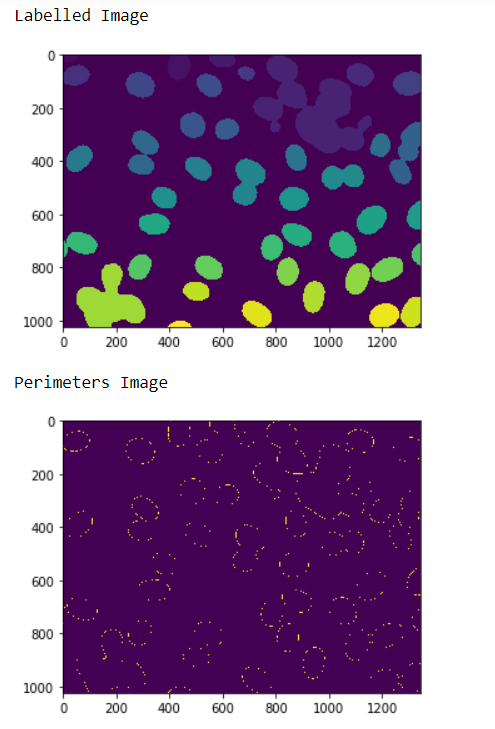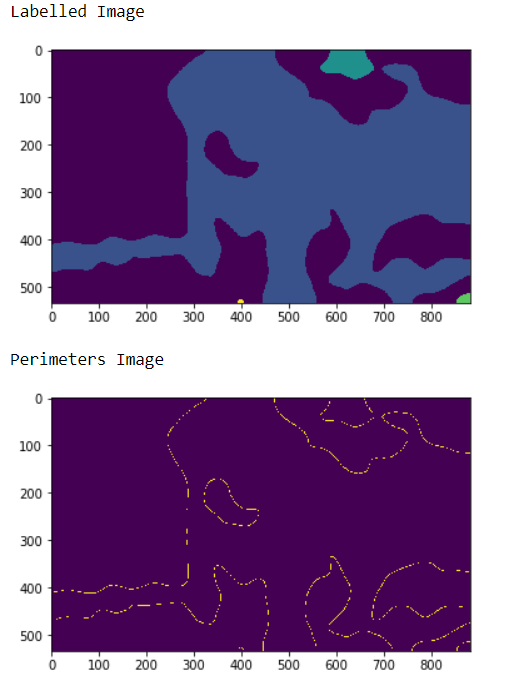Related Articles

# Mahotas – Perimeter of Objects in binary image

• Last Updated : 15 Apr, 2021

In this article we will see how we can get the perimeter of objects in binary images in mahotas. For this we are going to use the fluorescent microscopy image from a nuclear segmentation benchmark. We can get the image with the help of command given below

`mhotas.demos.nuclear_image()`

Below is the nuclear_imageA pixel is part of an object perimeter if its value is one and there is at least one zero-valued pixel in its neighborhood. By default the neighborhood of a pixel is 4 nearest pixels, but if we can set it to 8 then 8 nearest pixels will be considered.
In order to do this we will use mahotas.labelled.bwperim method

Syntax : mahotas.labeled.bwperim(image, n)
Argument : It takes numpy.ndarray object i.e labelled image preferred black and white and integer i.e nearest pixels which is optional
Return : It returns numpy.ndarray object i.e a boolean image

Note : The input of the this should should be the filtered image object which is labelled
In order to filter the image we will take the image object which is numpy.ndarray and filter it with the help of indexing, below is the command to do this

`image = image[:, :, 0]`

Example 1 :

## Python3

 `# importing required libraries``import` `mahotas``import` `numpy as np``from` `pylab ``import` `imshow, show``import` `os` `# loading nuclear image``f ``=` `mahotas.demos.load(``'nuclear'``)` `# setting filter to the image``f ``=` `f[:, :, ``0``]` `# setting gaussian filter``f ``=` `mahotas.gaussian_filter(f, ``4``)` `# setting threshold value``f ``=` `(f> f.mean())` `# creating a labelled image``labelled, n_nucleus ``=` `mahotas.label(f)`  `# showing the labelled image``print``(``"Labelled Image"``)``imshow(labelled)``show()` `# getting perimeters``relabelled ``=` `mahotas.labelled.bwperim(labelled)`  `# showing the image``print``(``"Perimeters Image"``)``imshow(relabelled)``show()`

Output :Example 2 :

## Python3

 `# importing required libraries``import` `numpy as np``import` `mahotas``from` `pylab ``import` `imshow, show`` ` `# loading image``img ``=` `mahotas.imread(``'dog_image.png'``)``   ` `# filtering the image``img ``=` `img[:, :, ``0``]``    ` `# setting gaussian filter``gaussian ``=` `mahotas.gaussian_filter(img, ``15``)`` ` `# setting threshold value``gaussian ``=` `(gaussian > gaussian.mean())`` ` `# creating a labelled image``labelled, n_nucleus ``=` `mahotas.label(gaussian)``  ` `print``(``"Labelled Image"``)``# showing the gaussian filter``imshow(labelled)``show()`` ` `# getting perimeters``relabeled ``=` `mahotas.labelled.bwperim(labelled, ``8``)`  `# showing the image``print``(``"Perimeters Image"``)``imshow(relabelled)``show()`

Output :Attention geek! Strengthen your foundations with the Python Programming Foundation Course and learn the basics.

To begin with, your interview preparations Enhance your Data Structures concepts with the Python DS Course. And to begin with your Machine Learning Journey, join the Machine Learning – Basic Level Course

My Personal Notes arrow_drop_up